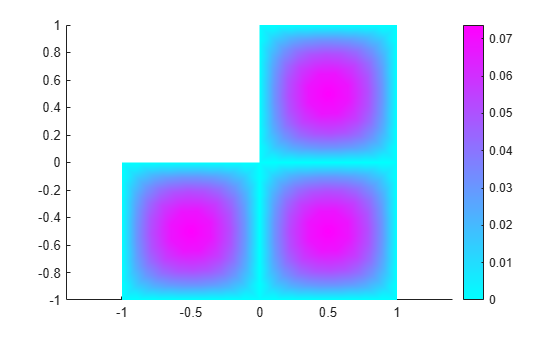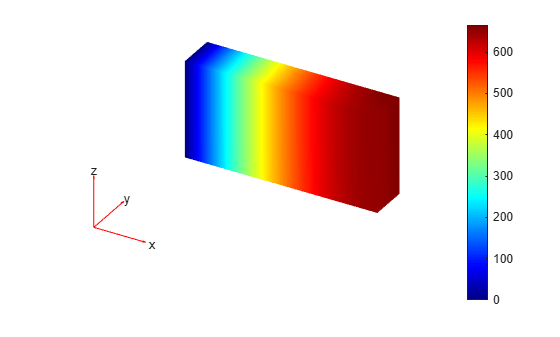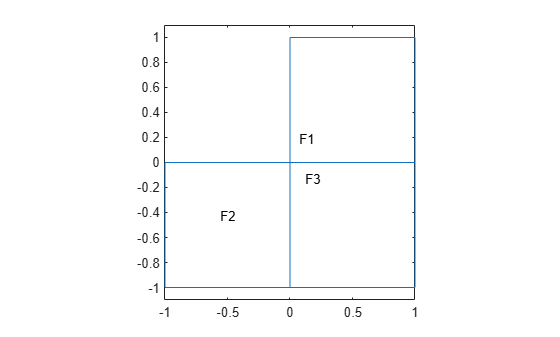# specifyCoefficients

Specify coefficients in a PDE model

## Syntax

``specifyCoefficients(model,Name,Value)``
``specifyCoefficients(model,Name,Value,RegionType,RegionID)``
``CA = specifyCoefficients(___)``

## Description

Coefficients of a PDE

`solvepde` solves PDEs of the form

`$m\frac{{\partial }^{2}u}{\partial {t}^{2}}+d\frac{\partial u}{\partial t}-\nabla ·\left(c\nabla u\right)+au=f$`

`solvepdeeig` solves PDE eigenvalue problems of the form

`$\begin{array}{l}-\nabla ·\left(c\nabla u\right)+au=\lambda du\\ \text{or}\\ -\nabla ·\left(c\nabla u\right)+au={\lambda }^{2}mu\end{array}$`

`specifyCoefficients` defines the coefficients m, d, c, a, and f in the PDE model.

example

````specifyCoefficients(model,Name,Value)` defines the specified coefficients in each `Name` to each associated `Value`, and includes them in `model`. You must specify all of these names: `m`, `d`, `c`, `a`, and `f`. This syntax applies coefficients to the entire geometry. NoteInclude geometry in `model` before using `specifyCoefficients`. ```

example

````specifyCoefficients(model,Name,Value,RegionType,RegionID)` assigns coefficients for a specified geometry region.```

example

````CA = specifyCoefficients(___)` returns a handle to the coefficient assignment object in `model`.```

## Examples

collapse all

Specify the coefficients for Poisson's equation $-\nabla \cdot \nabla u=1$.

`solvepde` addresses equations of the form

$m\frac{{\partial }^{2}u}{\partial {t}^{2}}+d\frac{\partial u}{\partial t}-\nabla \cdot \left(c\nabla u\right)+au=f$.

Therefore, the coefficients for Poisson's equation are $m=0$, $d=0$, $c=1$, $a=0$, $f=1$. Include these coefficients in a PDE model of the L-shaped membrane.

```model = createpde(); geometryFromEdges(model,@lshapeg); specifyCoefficients(model,'m',0,... 'd',0,... 'c',1,... 'a',0,... 'f',1);```

Specify zero Dirichlet boundary conditions, mesh the model, and solve the PDE.

```applyBoundaryCondition(model,'dirichlet','Edge',1:model.Geometry.NumEdges,'u',0); generateMesh(model,'Hmax',0.25); results = solvepde(model);```

View the solution.

`pdeplot(model,'XYData',results.NodalSolution)`Specify coefficients for Poisson's equation in 3-D with a nonconstant source term, and obtain the coefficient object.

The equation coefficients are $m=0$, $d=0$, $c=1$, $a=0$. For the nonconstant source term, take $f={y}^{2}\mathrm{tanh}\left(z\right)/1000$.

`f = @(location,state)location.y.^2.*tanh(location.z)/1000;`

Set the coefficients in a 3-D rectangular block geometry.

```model = createpde(); importGeometry(model,'Block.stl'); CA = specifyCoefficients(model,'m',0,... 'd',0,... 'c',1,... 'a',0,... 'f',f)```
```CA = CoefficientAssignment with properties: RegionType: 'cell' RegionID: 1 m: 0 d: 0 c: 1 a: 0 f: @(location,state)location.y.^2.*tanh(location.z)/1000 ```

Set zero Dirichlet conditions on face 1, mesh the geometry, and solve the PDE.

```applyBoundaryCondition(model,'dirichlet','Face',1,'u',0); generateMesh(model); results = solvepde(model);```

View the solution on the surface.

`pdeplot3D(model,'ColorMapData',results.NodalSolution)`Create a scalar PDE model with the L-shaped membrane as the geometry. Plot the geometry and subdomain labels.

```model = createpde(); geometryFromEdges(model,@lshapeg); pdegplot(model,'FaceLabels','on') axis equal ylim([-1.1,1.1])```Set the `c` coefficient to 1 in all domains, but the `f` coefficient to 1 in subdomain 1, 5 in subdomain 2, and -8 in subdomain 3. Set all other coefficients to 0.

```specifyCoefficients(model,'m',0,'d',0,'c',1,'a',0,'f',1,'Face',1); specifyCoefficients(model,'m',0,'d',0,'c',1,'a',0,'f',5,'Face',2); specifyCoefficients(model,'m',0,'d',0,'c',1,'a',0,'f',-8,'Face',3);```

Set zero Dirichlet boundary conditions to all edges. Create a mesh, solve the PDE, and plot the result.

```applyBoundaryCondition(model,'dirichlet','Edge',1:model.Geometry.NumEdges,'u',0); generateMesh(model,'Hmax',0.25); results = solvepde(model); pdeplot(model,'XYData',results.NodalSolution)```## Input Arguments

collapse all

PDE model, specified as a `PDEModel` object.

Example: `model = createpde`

### Name-Value Pair Arguments

Example: `specifyCoefficients(model,'m',0,'d',0,'c',1,'a',0,'f',@fcoeff)`

### Note

You must specify all of these names: `m`, `d`, `c`, `a`, and `f`.

Second-order time derivative coefficient, specified as a scalar, column vector, or function handle. For details on the sizes, and for details of the function handle form of the coefficient, see m, d, or a Coefficient for specifyCoefficients.

Specify `0` if the term is not part of your problem.

Example: `specifyCoefficients('m',@mcoef,'d',0,'c',1,'a',0,'f',1,'Face',1:4)`

Data Types: `double` | `function_handle`
Complex Number Support: Yes

First-order time derivative coefficient, specified as a scalar, column vector, or function handle. For details on the sizes, and for details of the function handle form of the coefficient, see m, d, or a Coefficient for specifyCoefficients.

### Note

If the `m` coefficient is nonzero, `d` must be `0` or a matrix, and not a function handle. See d Coefficient When m is Nonzero.

Specify `0` if the term is not part of your problem.

Example: `specifyCoefficients('m',0,'d',@dcoef,'c',1,'a',0,'f',1,'Face',1:4)`

Data Types: `double` | `function_handle`
Complex Number Support: Yes

Second-order space derivative coefficient, specified as a scalar, column vector, or function handle. For details on the sizes, and for details of the function handle form of the coefficient, see c Coefficient for specifyCoefficients.

Example: `specifyCoefficients('m',0,'d',0,'c',@ccoef,'a',0,'f',1,'Face',1:4)`

Data Types: `double` | `function_handle`
Complex Number Support: Yes

Solution multiplier coefficient, specified as a scalar, column vector, or function handle. For details on the sizes, and for details of the function handle form of the coefficient, see m, d, or a Coefficient for specifyCoefficients.

Specify `0` if the term is not part of your problem.

Example: `specifyCoefficients('m',0,'d',0,'c',1,'a',@acoef,'f',1,'Face',1:4)`

Data Types: `double` | `function_handle`
Complex Number Support: Yes

Source coefficient, specified as a scalar, column vector, or function handle. For details on the sizes, and for details of the function handle form of the coefficient, see f Coefficient for specifyCoefficients.

Specify `0` if the term is not part of your problem.

Example: `specifyCoefficients('m',0,'d',0,'c',1,'a',0,'f',@fcoeff,'Face',1:4)`

Data Types: `double` | `function_handle`
Complex Number Support: Yes

Geometric region type, specified as `'Face'` or `'Cell'`.

Example: `specifyCoefficients('m',0,'d',0,'c',1,'a',0,'f',10,'Cell',2)`

Data Types: `char` | `string`

Geometric region ID, specified as a vector of positive integers. Find the region IDs by using `pdegplot`.

Example: `specifyCoefficients('m',0,'d',0,'c',1,'a',0,'f',10,'Cell',1:3)`

Data Types: `double`

## Output Arguments

collapse all

Coefficient assignment, returned as a CoefficientAssignment Properties object.

collapse all

### `d` Coefficient When `m` is Nonzero

The `d` coefficient takes a special matrix form when `m` is nonzero. You must specify `d` as a matrix of a particular size, and not as a function handle.

`d` represents a damping coefficient in the case of nonzero `m`. To specify `d`, perform these two steps:

1. Call `results = assembleFEMatrices(...)` for the problem with your original coefficients and using `d` = 0. Use the default `'none'` method for `assembleFEMatrices`.

2. Take the `d` coefficient as a matrix of size `results.M`. Generally, `d` is either proportional to `results.M`, or is a linear combination of `results.M` and `results.K`.

## Tips

• For eigenvalue equations, the coefficients cannot depend on the solution `u` or its gradient.